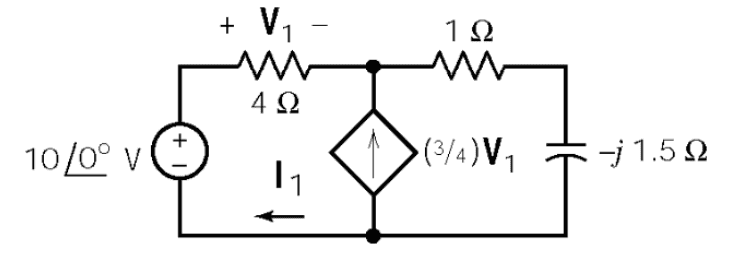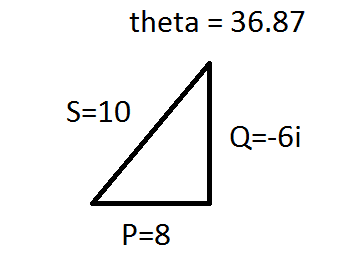# Mesh analysis with dependent voltage source

## Homework Statement

Find the:
-Complex power delivered by source
-Is the power factor leading, lagging, or in unity?[/B]KVL/KCL

## The Attempt at a Solution

I want to solve this via mesh analysis to get currents.
Mesh1: -10V + V1 + 0.75V1 = 0
V1 = 40/7V
4I1 = 40/7
I1 = 10/7 A

Mesh2: -0.75V1 = I2(1-j1.5) = 0
I2(1-j1.5) = 0.75 * 40/7
I2 = 30/(7(1-j1.5))

Did I do this right?

gneill
Mentor
The controlled source depicted is a current source, not a voltage source. So either employ a supermesh or introduce another variable to represent the potential across it (and you'll need another equation so that you have as many equations as variables in your equation set).

The controlled source depicted is a current source, not a voltage source. So either employ a supermesh or introduce another variable to represent the potential across it (and you'll need another equation so that you have as many equations as variables in your equation set).
-10V + 4(I1) + I2(1 - j1.5) = 0
I2 = 3/4V1 + I1
V1 = 4(I1)

Are these correct?

gneill
Mentor
Yes, those look good.

Yes, those look good.
i1 = 0.8 + 0.6i
i2 = 3.2 + 2.4i
correct?

gneill
Mentor
That looks good, too.

That looks good, too.
How would I find complex power supplied by the source?

gneill
Mentor
How would I find complex power supplied by the source?
Use the current supplied by the source and the source voltage.

Use the current supplied by the source and the source voltage.
Is current supplied by source I1 - 3/4V1?
How would I find the power factor?

gneill
Mentor
Presumably the source referred to is the 10 V voltage source. It supplies just I1.

For the power factor you need to look at the power triangle. The complex power will give you the two "legs" of the triangle.

Presumably the source referred to is the 10 V voltage source. It supplies just I1.

For the power factor you need to look at the power triangle. The complex power will give you the two "legs" of the triangle.
So complex power = 10 * I* = 10 * (0.8 - 0.6i)?
S = 8-6i
Power factor = P/S = 8/10 = 0.8 leading? (Q<0)
Does this triangle look right?cnh1995
Homework Helper
Gold Member
So complex power = 10 * I* = 10 * (0.8 - 0.6i)?
S = 8-6i
Power factor = P/S = 8/10 = 0.8 leading?
Yes. Also, write 'j' instead of 'i' in the imaginary part of the complex form (e.g
S=8-j6). That's a convention followed in order to avoid confusion with the symbol 'I' for current.

Yes. Also, write 'j' instead of 'i' in the imaginary part of the complex form (e.g
S=8-j6). That's a convention followed in order to avoid confusion with the symbol 'I' for current.
Can (should) I convert to VA form? (sqrt(P^2+Q^2))

cnh1995
Homework Helper
Gold Member
Can (should) I convert to VA form? (sqrt(P^2+Q^2))
Not necessary if apparent power is not asked. You can keep it in the complex form.